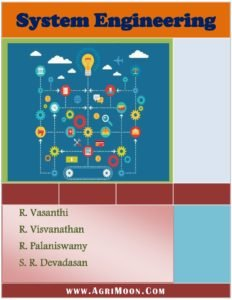You are here
Home > Book > System Engineering PDF Book

# System Engineering PDF Book

Book Detail: System EngineeringLanguage: English

Pages: 273

Author: Mrs. R. Vasanthi, Dr. R. Visvanathan, Dr. R. Palaniswamy, Dr. S. R. Devadasan

Price: FreeOutlines of System Engineering

Module 1. Systems concept

Lesson 1. Systems concept: operations management

Lesson 2. Systems concept: managerial policy and decision making

Lesson 3. Systems concept : methodology and models

Module 2. Requirements for linear programming problems

Lesson 4. Origin and development of or

Lesson 5. Methodology- uses and limitations

Lesson 6. Linear programming – basic ideas

Module 3. Mathematical formulation of linear programming problems and its graphical solution

Lesson 7. Mathematical formulation of the problem

Lesson 8. Graphical method i

Lesson 9. Graphical method ii – special cases

Module 5. Simplex method, degeneracy and duality in linear programming

Lesson 10. Simplex method

Lesson 11. Simplex method problems

Lesson 12. Degeneracy

Lesson 13. Duality in linear programming

Lesson 14. Simplex method –problems

Module 6. Artificial variable techniques- big m method, two phase methods

Lesson 15. Artificial variable techniques- big m method

Lesson 16. Big m method – problems

Lesson 17. Big m method- special case

Lesson 18. Artificial variable techniques- two phase method

Module 7.

Lesson 19. Introduction to models

Lesson 20. Growth models

Module 8.

Lesson 21. Introduction to agricultural system

Lesson 22. Linear growth model

Lesson 23. Logistic growth model

Lesson 24. Richard’s growth model

Module 9. Cost analysis

Lesson 25. Cost analysis: cost of operation / production

Lesson 26. Investment analysis

Lesson 27. Inventory control

Module 10. Transporatation problems

Lesson 28. Transportation problems

Lesson 29. Transportation problems

Lesson 30. Transportation problems

Module 11. Assignment problems

Lesson 31. Assignment problems – introduction

Lesson 32. Assignment problems – solutions of the assignment problems

Lesson 33. Assignment problems – assignment algorithm

Module 12. Waiting line problems

Lesson 34. Introduction to queuing theory

Lesson 35. Characteristics of queueing systems

Lesson 36. Classification of queuing models and their solutions

Lesson 37 solved examples

Module 13. Network scheduling by PERT / CPM

Lesson 38. Network analysis

Lesson 39. Construction

Lesson 40. Critical path method

Lesson 41. Programme evaluation review technique: (pert)

Lesson 42. Network problems

Module 14. Resource analysis in network scheduling

Lesson 43. Project cost and resource leveling

Lesson 44. Time-cost optimization

Lesson 45. Time cost optimization- problems

Disclaimer:The information on this website does not warrant or assume any legal liability or responsibility for the accuracy, completeness or usefulness of the courseware contents.

The contents are provided free for noncommercial purpose such as teaching, training, research, extension and self learning.

If you want share any article related Agriculture with us than send at info@agrimoon.com with your contact detail.

## 4 thoughts on “System Engineering PDF Book”

1.Abhishek Anand says:

Very Nice Books

2.James Flomo Gaydaybu says:

Good book!

This site uses Akismet to reduce spam. Learn how your comment data is processed.